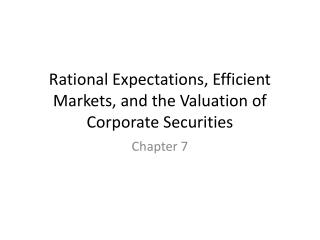# Rational Expectations, Efficient Markets, and the Valuation of Corporate Securities - PowerPoint PPT PresentationDownload PresentationRational Expectations, Efficient Markets, and the Valuation of Corporate Securities

Rational Expectations, Efficient Markets, and the Valuation of Corporate SecuritiesDownload Presentation## Rational Expectations, Efficient Markets, and the Valuation of Corporate Securities

- - - - - - - - - - - - - - - - - - - - - - - - - - - E N D - - - - - - - - - - - - - - - - - - - - - - - - - - -
##### Presentation Transcript

1. Rational Expectations, Efficient Markets, and the Valuation of Corporate Securities Chapter 7

2. Learning Objectives • Define rational expectations • Explain how corporate equities are valued under the assumption of rational expectations • Write down and apply the equation for the Gordon growth model • Distinguish between the three forms of market efficiency and relate to fundamental and technical analysis • Explain how bubbles can form

3. Rational Expectation • expectation based on all available information • an educated guess • example: estimating travel time • not influenced by psychological bias

4. Intrinsic (or fundamental) value of a financial instrument The rational expectation of the value Present value of all future cash flows

5. Future cash flows for an equity security Dividends: share of profit of company distributed to the owners Usually paid on a quarterly basis (every 3 months). Let Et represent the expected dividend at time t

6. What if a company does not pay dividends? Estimate the period in which it will begin to pay them

7. Recall: Present Value Formula PV present value FV future value iperiodic interest rate in decimal form n number of periods

8. Rate of discount For equities instead of using the interest rate, use the required rate of return, k k reflects the risk that dividends will not be as high as expected

9. Value of Corporate Equity Present value each dividend using k as the rate of discount, and add them all together

10. Gordon Growth Model Assume dividends grow at a constant rate g So, Et = Et-1 (1+g) If the most recent dividend (E0 ) was \$100 and dividends are expected to grow at an annualized rate of 8%, then this quarter’s dividend should be \$102 And next quarter’s dividend will be \$104.04

11. Gordon Growth Model Present value each dividend using k as the rate of discount, and add them all together

12. Gordon Growth Model Using the formula for an infinite series the present value formula simplifies down to:

13. Efficient Markets Hypothesis The prices of equities reflect their intrinsic (or fundamental) values … … that is the present value of future dividends.

14. Three Forms of Market Efficiency • Weak: past prices no use • Semi-strong: publicly available info no use • Strong: even inside info no use

15. Implication of Market Efficiency • Best to invest in low cost index funds

16. Fundamental vs Technical Analysis

17. Anomalies • Small firm effect • Mean reversion • January effect

18. Behavioral Finance Studies both rational, and irrational, expectations. http://www.nbr.com/videos/video/1397919189001/dan-ariely-on-framing-and-investment-decisions-may-24-2010#.UG0Enxgx_2s To learn more about behavioral economics, see http://danariely.com/

19. Bubbles • Prices going above their intrinsic value • Hard to see when they are happening but obvious after they burst

20. 1636: One tulip cost 10X the salary of a skilled craftsman.